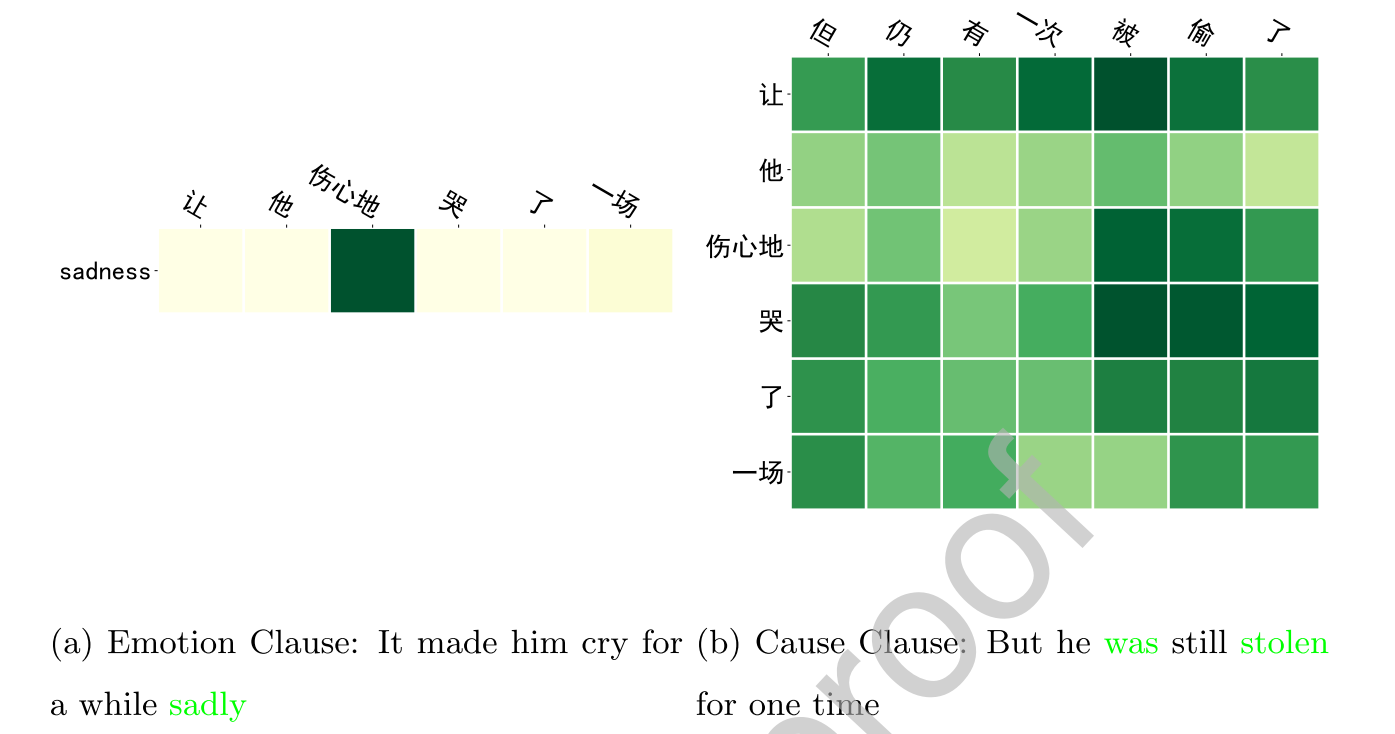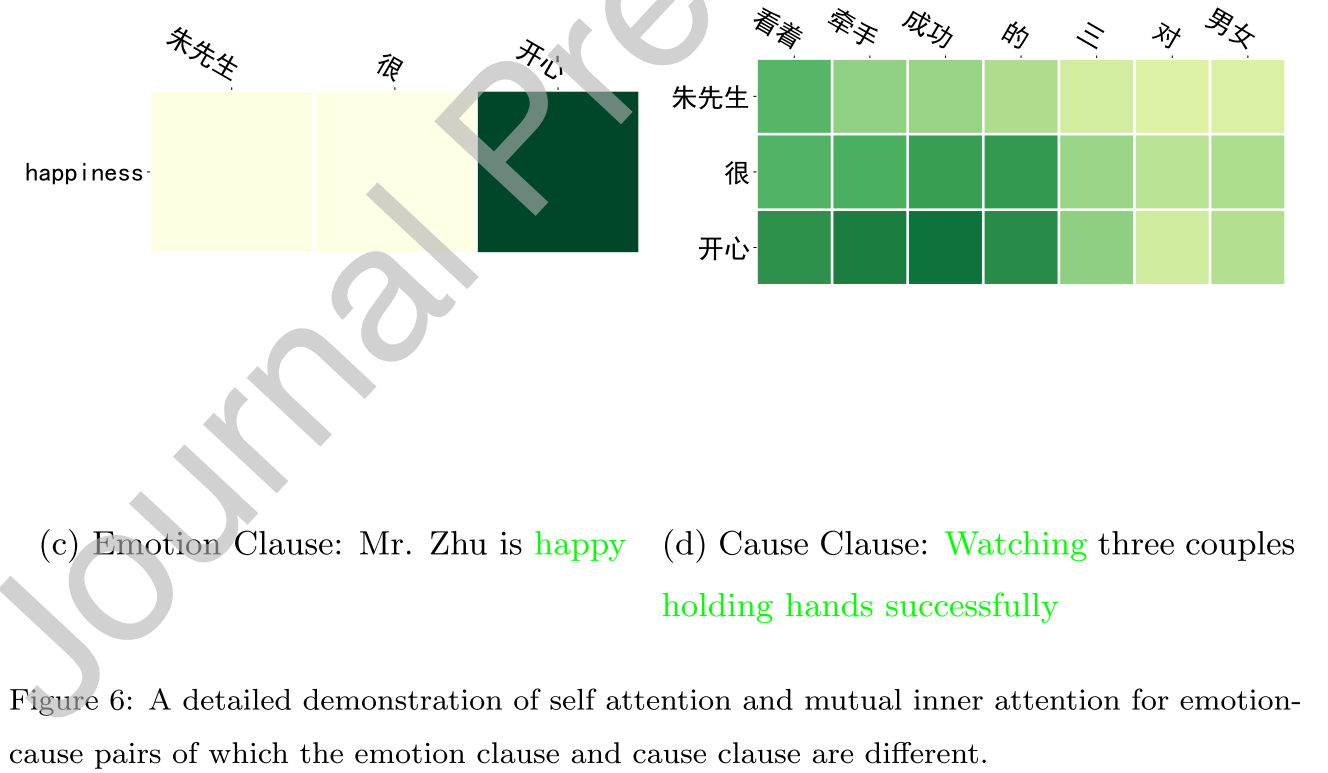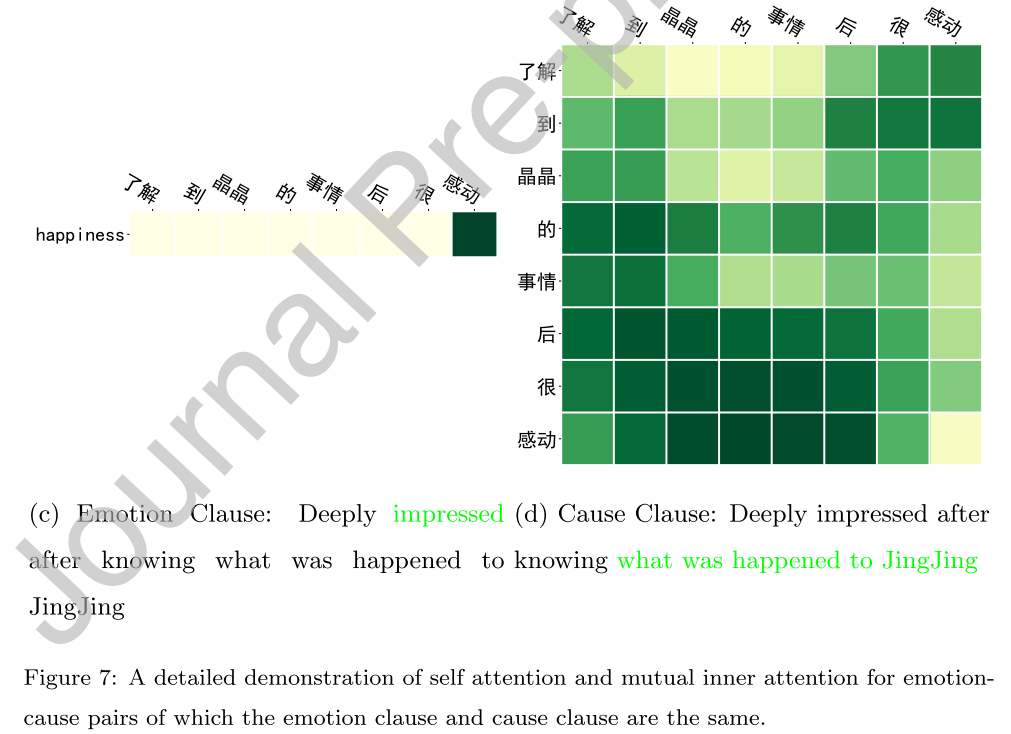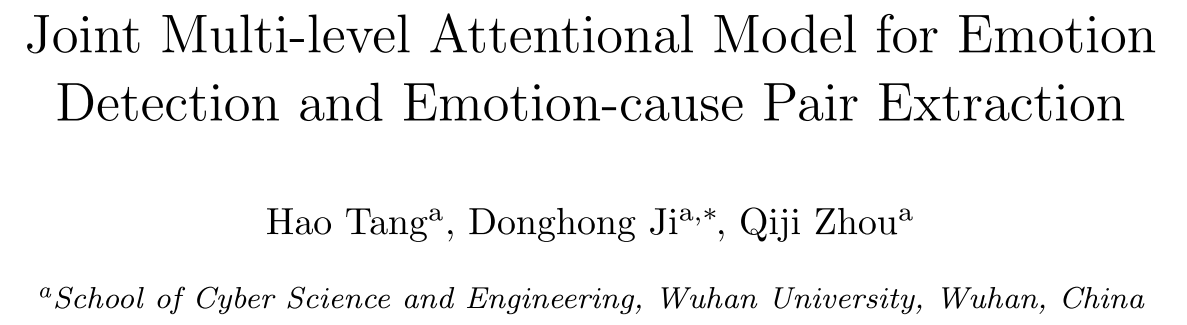# 1. 摘要和介绍

ECE任务旨在推断出文本中的情感表示，这个任务首先被当做词级别的序列标注问题；后来Gui et al.(2016)又重新设计了用于原因子句检测的ECE任务，并且将该任务建模为子句级别的分类问题。再后来，Xia et al.(2019)认为在文档级的表示中，情感子句和它对应的原因子句之间存在隐式联系，而且ECE任务需要先标注情感子句，这不够实用，所以又提出了新任务emotion-cause pair extraction(ECPE)，旨在预测出文档中的情感子句-原因子句对，即子句级别的对分类问题。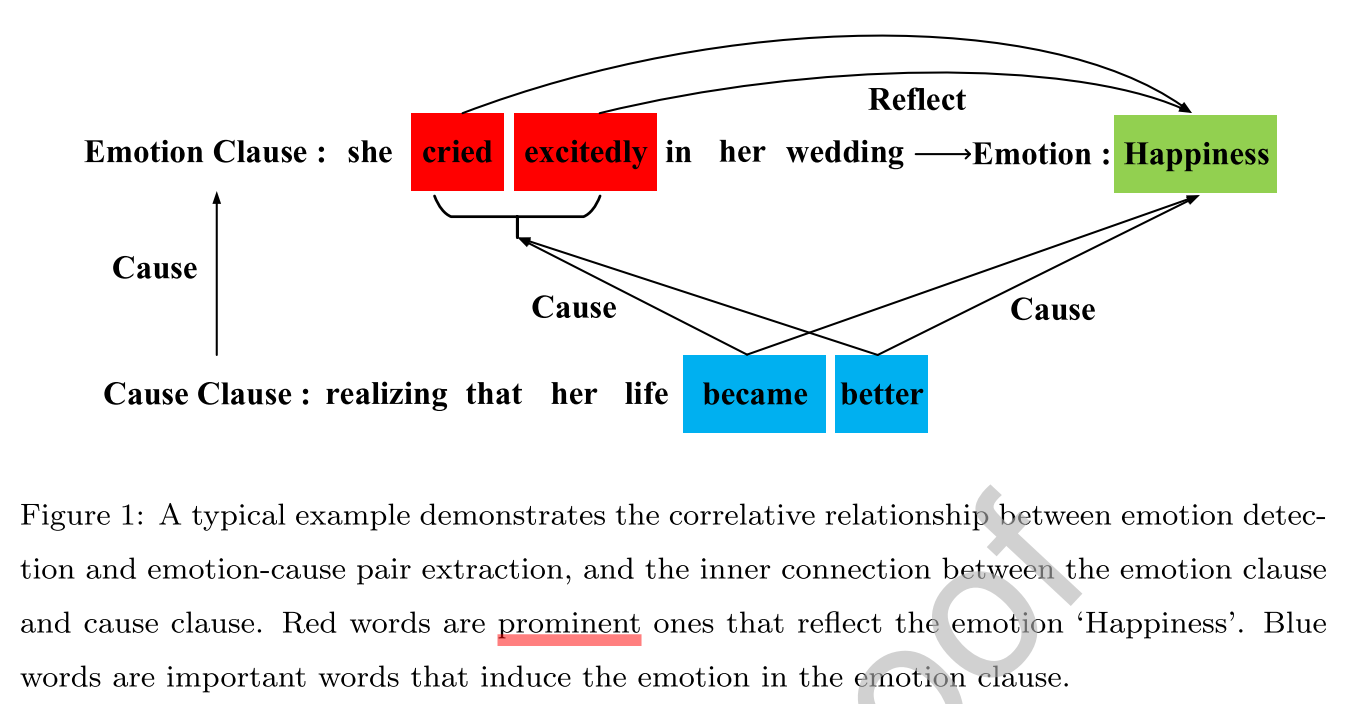1. ECPE中所有的情感子句肯定都有自己的情感类别（比如开心、悲伤等），这意味着ECPE中的情感子句在ED中也是情感子句；
2. 原因子句也与给定的情感有关，不同的语义片段会导致不同的情感。比如说图中原因子句中的became better也有助于检测情感子句的情感类别——Happiness。

# 3. 准备工作

## 3.1 ECE的基本分层模型

ECE的基本分层模型包含三个组件：子句编码器，上下文编码器，CRF。

• 子句编码器用来编码并且表示子句信息；

• 上下文编码器在子句编码器的输出上，计算子句级的嵌入；

• CRF层的目的是作为一种后处理方法来优化标签预测。

# 4. 模型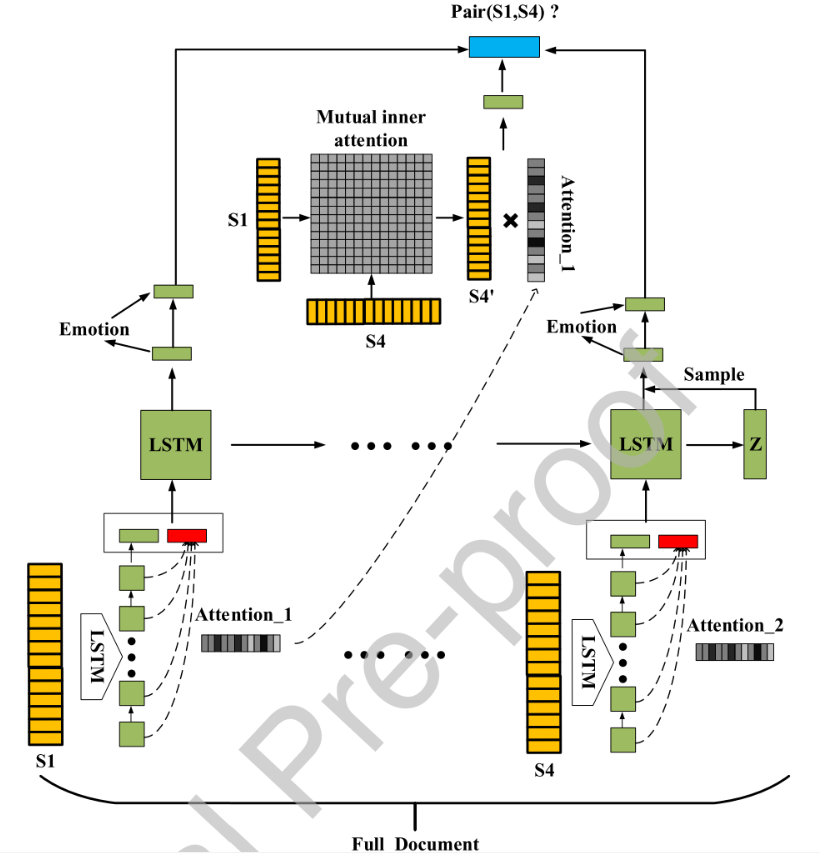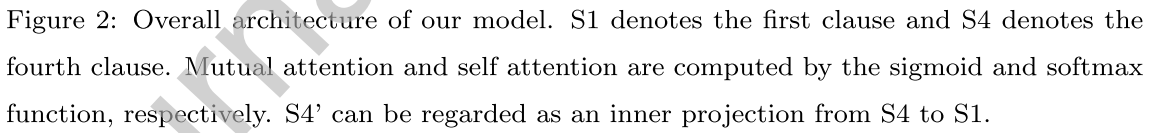## 4.1 BERT子句编码器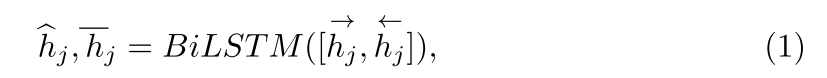$h_j = \{h_{j,0}, h_{j,1},....\}$是第j个子句的embedding，$\hat{h}_j=\{\hat{h}_{j,0},.... \}$是BiLSTM的输出，$\bar{h}_j$是BiLSTM的最后隐藏状态，j是子句的下标。

## 4.2 Self Attention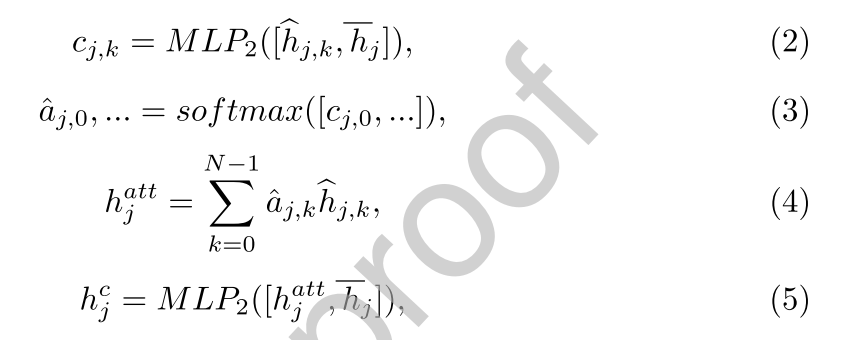$\hat{h}_{j,k}$是第j个子句第k个词的隐藏态表示，$\bar{h}_j$是第j个子句的隐藏态表示。即前者是词表示，后者是句表示。$MLP_2$是两层的多层感知机，且带有激活函数tanh。

（2）式得到了$c_{j,0},c_{j,1},c_{j,2},....$之后，传入（3）式，得到第j个子句中第i个词的注意力权重$\hat{a}_{j, i}$

$h_j^{att}$是带权和，也就是过了self attention之后的第j个子句的新向量表示，随后作者又过了一个两层的感知机，得到第j个子句的最终向量表示$h_j^c$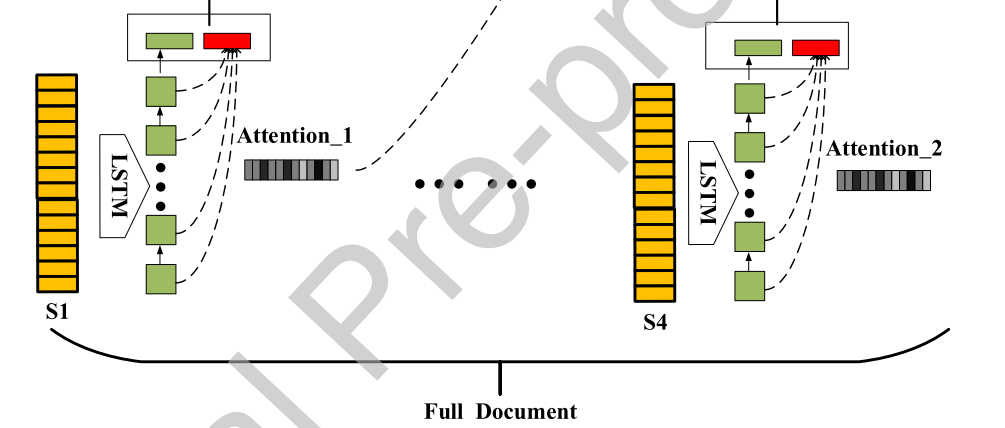4.1, 4.2节就对应该图部分，红色框就表示$\bar{h}_j$

## 4.3 上下文编码器和潜在变量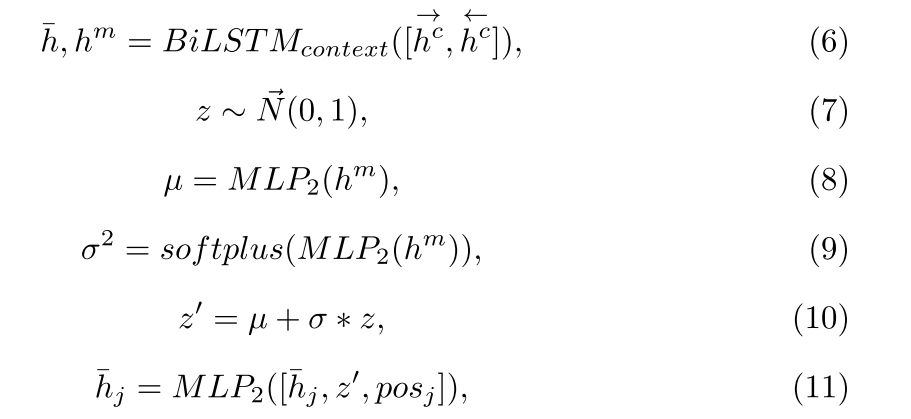$h^c$是4.2节得到的子句的向量表示，将所有子句的向量表示送入$BiLSTM_{context}$，得到两个输出。

$\bar{h}=\{\bar{h}_0,\bar{h}_1,... \}$是每个子句的隐藏状态向量表示的集合，$h^m$是BiLSTM的最后隐藏状态。$softplus$是非线性函数，确保输出值大于0。

$pos_j$是下标为j的位置嵌入。最后得到的$\bar{h}_j$就是第j个子句的新向量表示。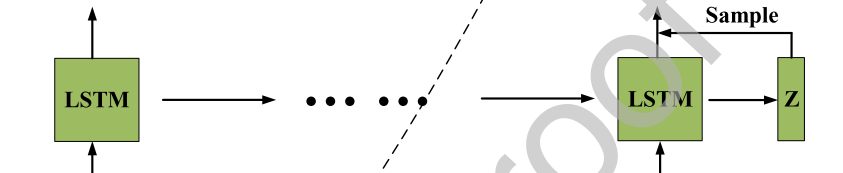## 4.4 Mutual Attention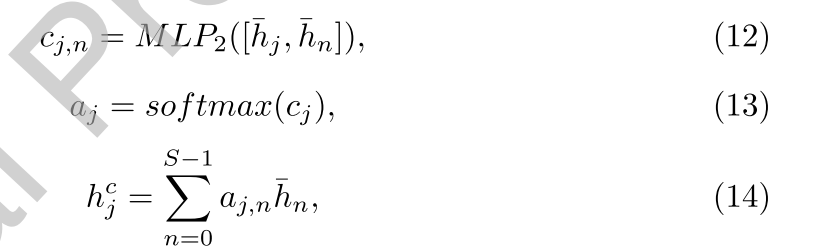$\bar{h}_j$就是第j个子句的向量表示,$\bar{h}_n$就是第n个子句的向量表示。$h_j^c$是经过mutual attention后第j个子句的嵌入表示，$S$是文档中的子句数。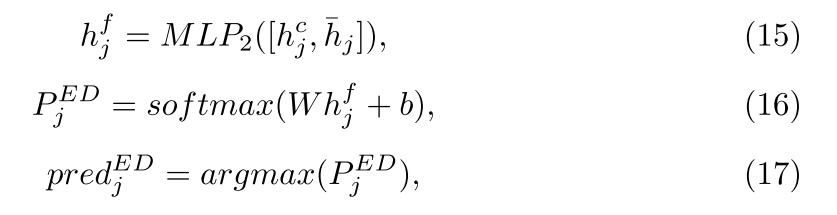$pred_j^{ED}$后面还会用于ECPE任务。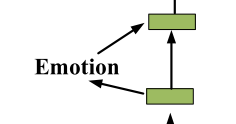4.4节对应上图，Emotion就是$pred_j^{ED}$

## 4.5 多层次注意力模块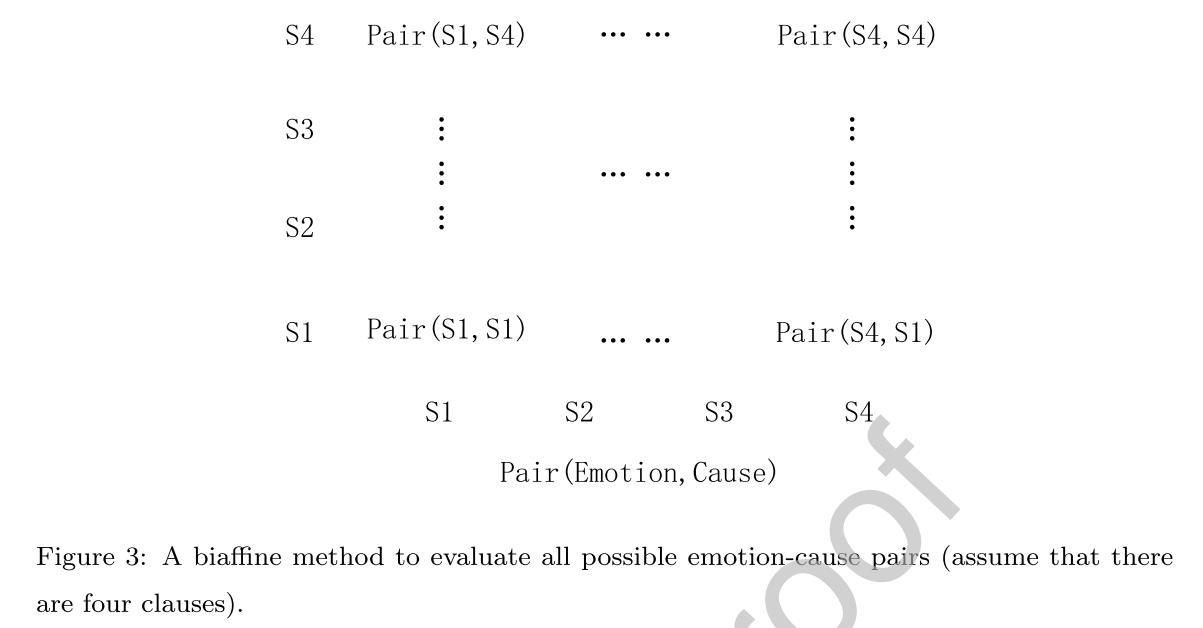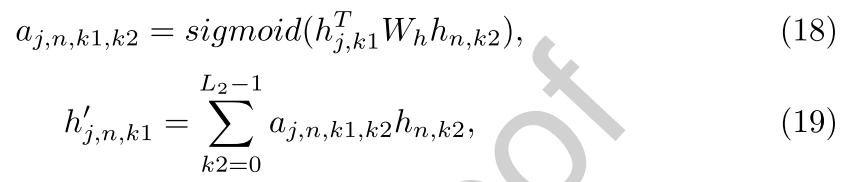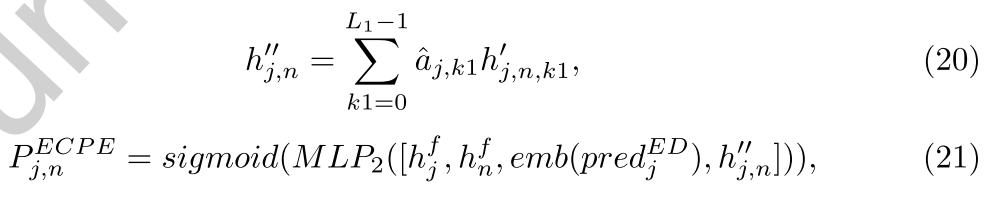$j,n$是情感和原因子句的下标，$k1, k2$是情感和原因子句中单词的下标。所以$h_{j,k1}$就表示第j个子句中的第k1个词的向量表示，$h_{n,k2}$就表示第n个文档中的第k2个词的向量表示。$L1,L2$分别表示第$j, n$个子句的长度。

$\hat{a}_{j, k_1}$是由（3）式self attention得到的，反映了形成最终情感子句的第k1个单词的贡献价值。

$h''_{j,n}$是一个对齐向量，可以看做是原因子句到情感子句的映射。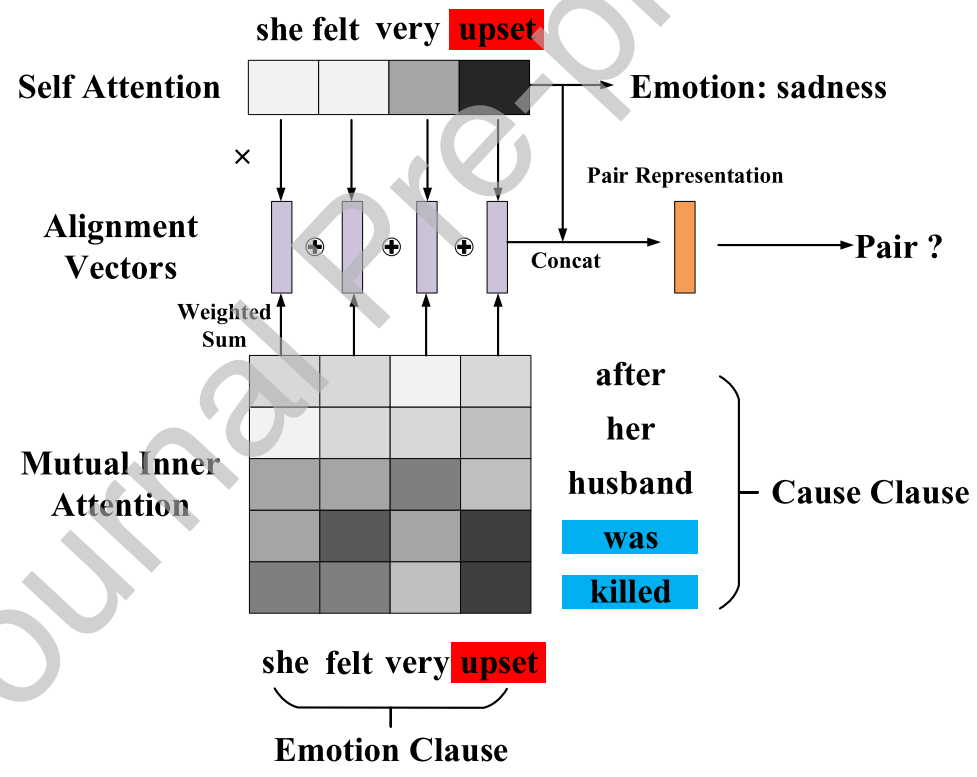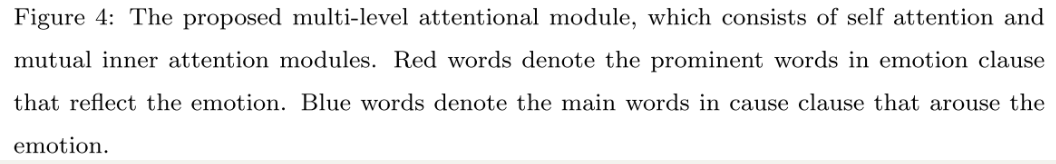## 4.6 损失函数

KL散度公式如下：

$D_{KL}(p||q) = \sum_{i=1}^np(x)log\frac{p(x)}{q(x)}$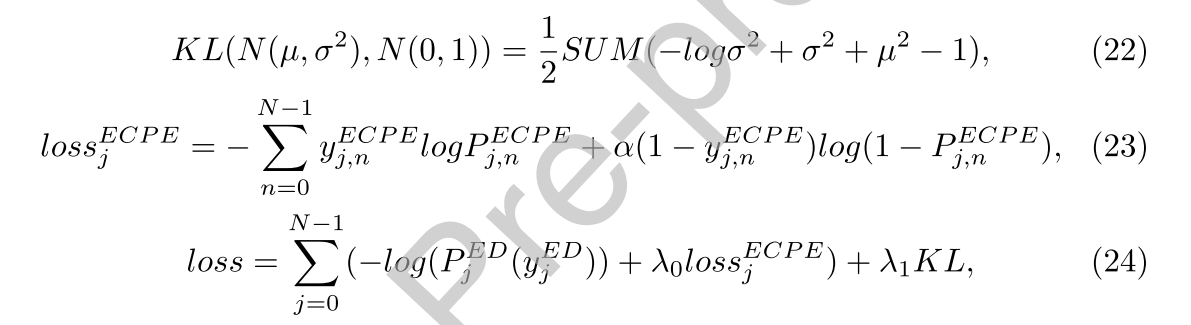$N$是文档的总子句数，$\mu, \sigma$都是向量，$KL$值是标量。

$y_j^{ED}$是ED任务的第j个子句的情感标签，$y_{j,n}^{ECPE}$是对$pair(j,n)$的golden标签，$j,n$是对中两个子句的下标，若对是情感-原因对，$y^{ECPE}=1$，否则为0。

golden应该就是ground-truth的意思吧…

## 4.7 只用于ECE任务的Mutual Inner Attention

4.4节中（12）-（14）三个等式用以下等式替换：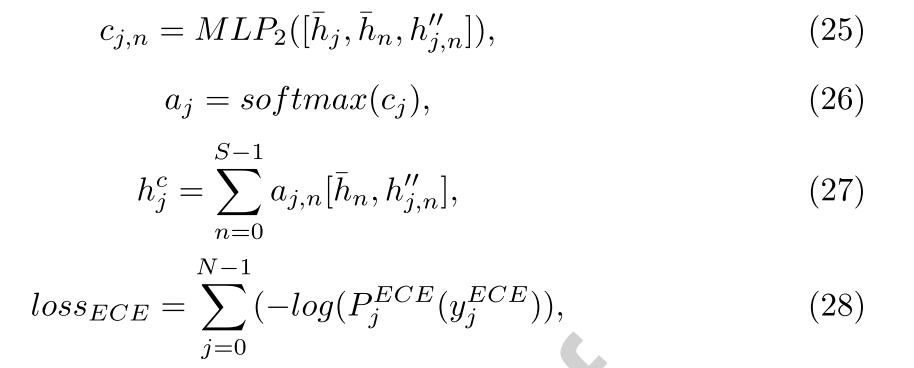ECE任务的标签只有两个类型：cause or non-cause。

# 5. 实验

ECE数据集和ECPE数据集

## 5.4 评估标准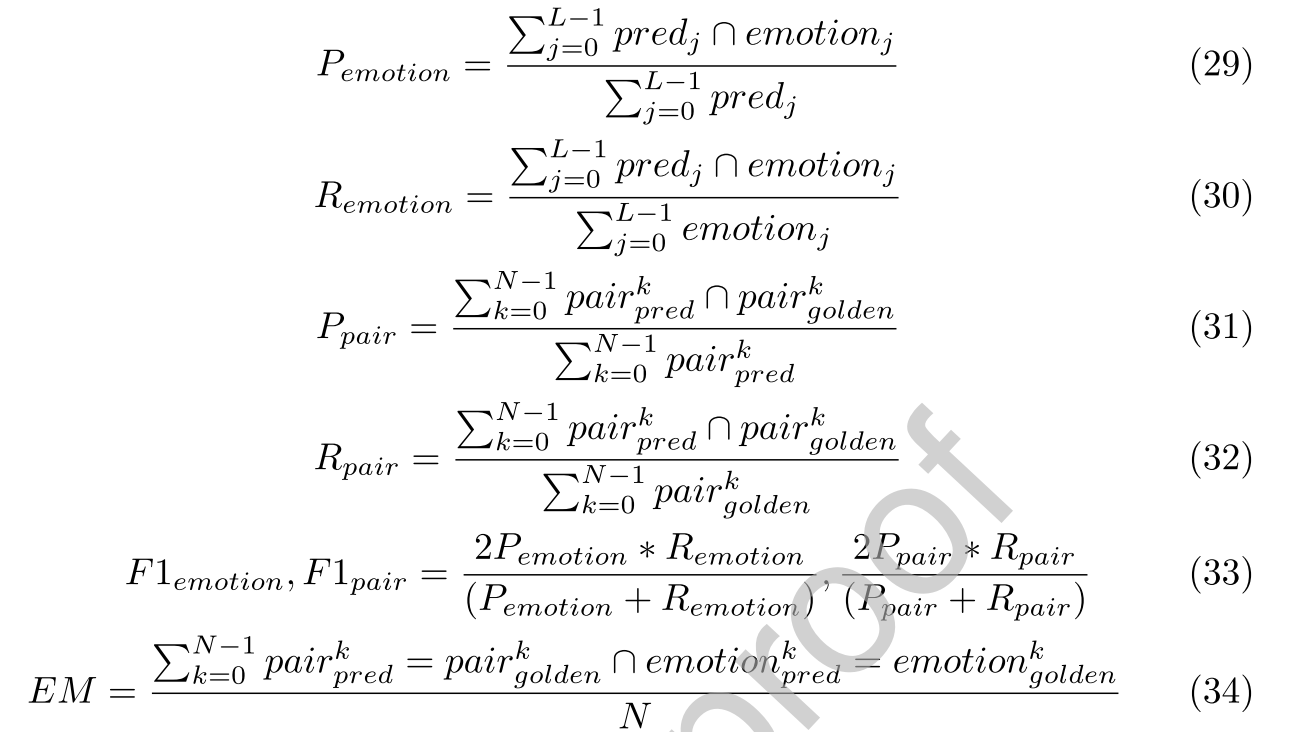## 5.5 主要结果

### 5.5.1 ECE任务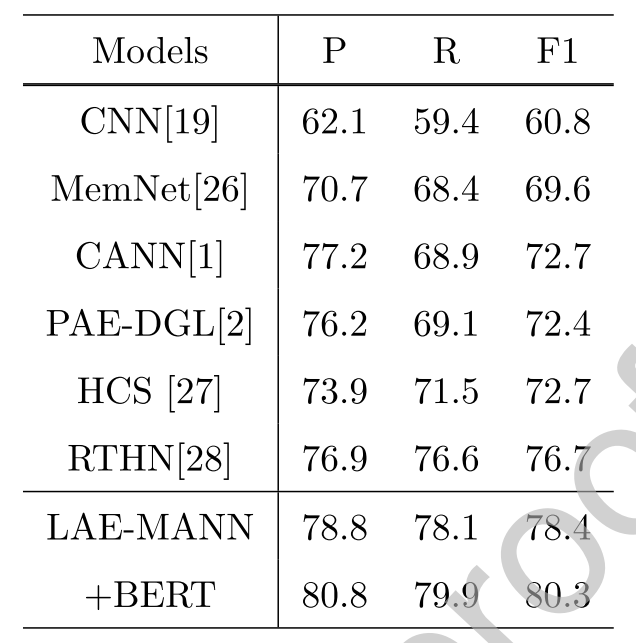### 5.5.2 ED和ECPE任务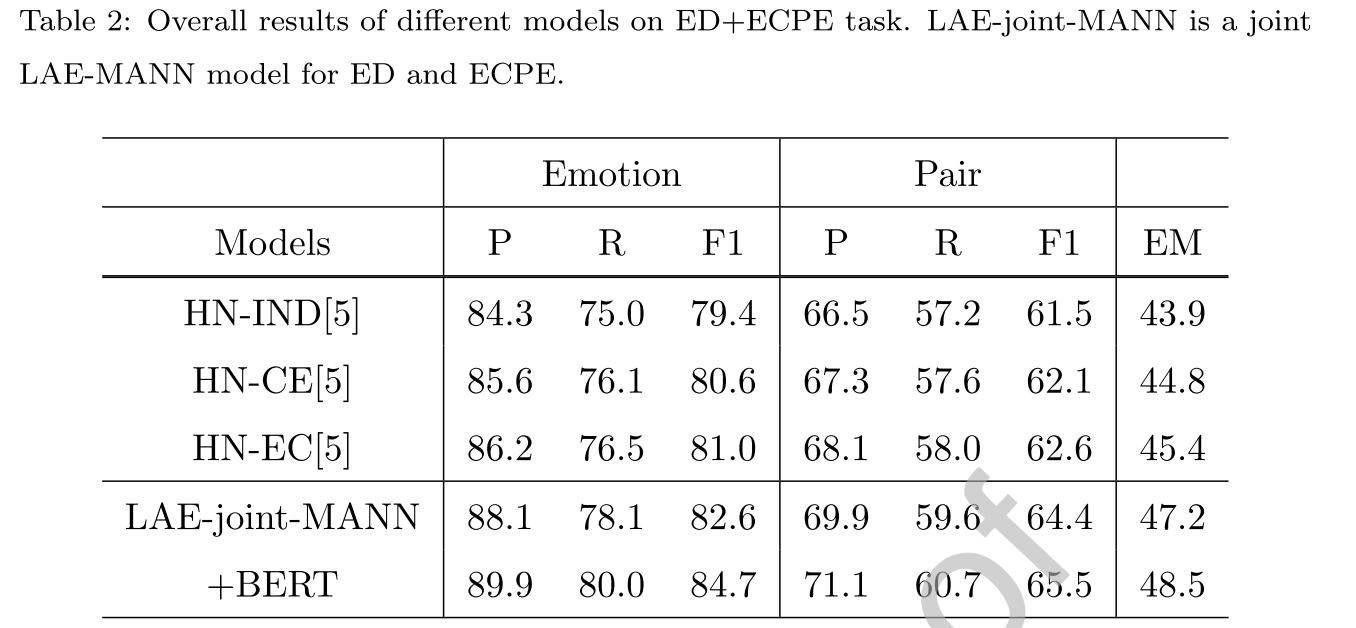## 5.6 消融实验

SA表示self attention；MA表示mutual attention；LV表示latent variable；MIA表示mutual inner attention；Joint表示模型是否被联合训练；EL表示模型是否使用ED任务的预测结果。

ECE任务中是没有Joint和EL的。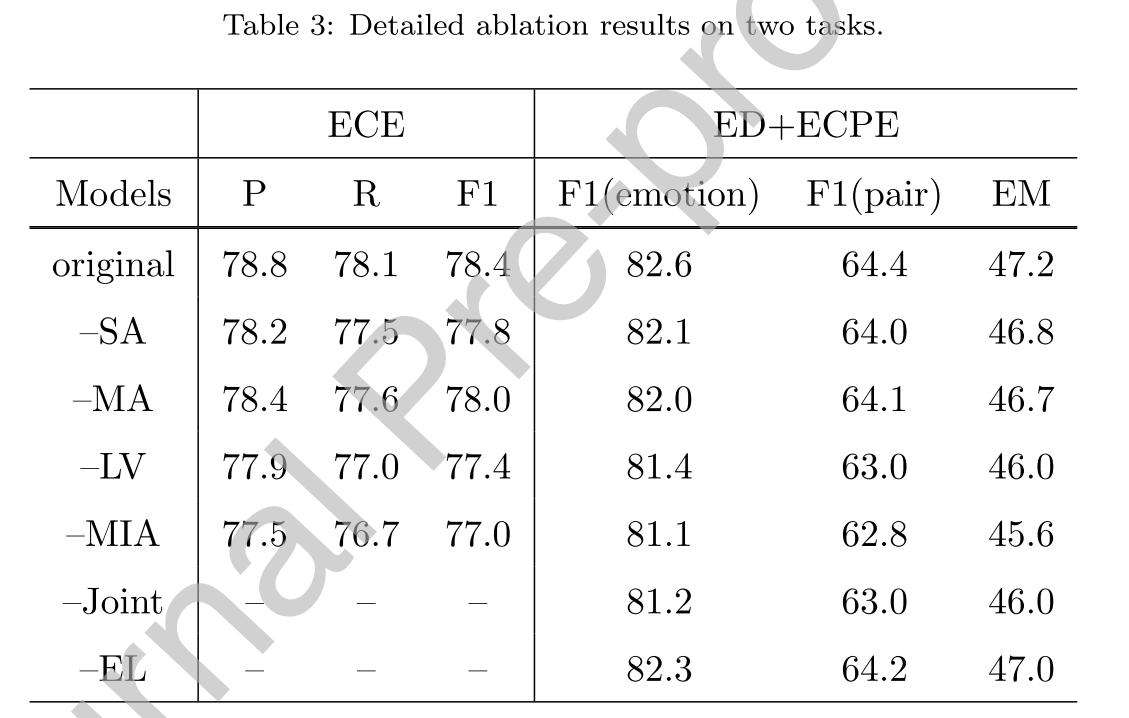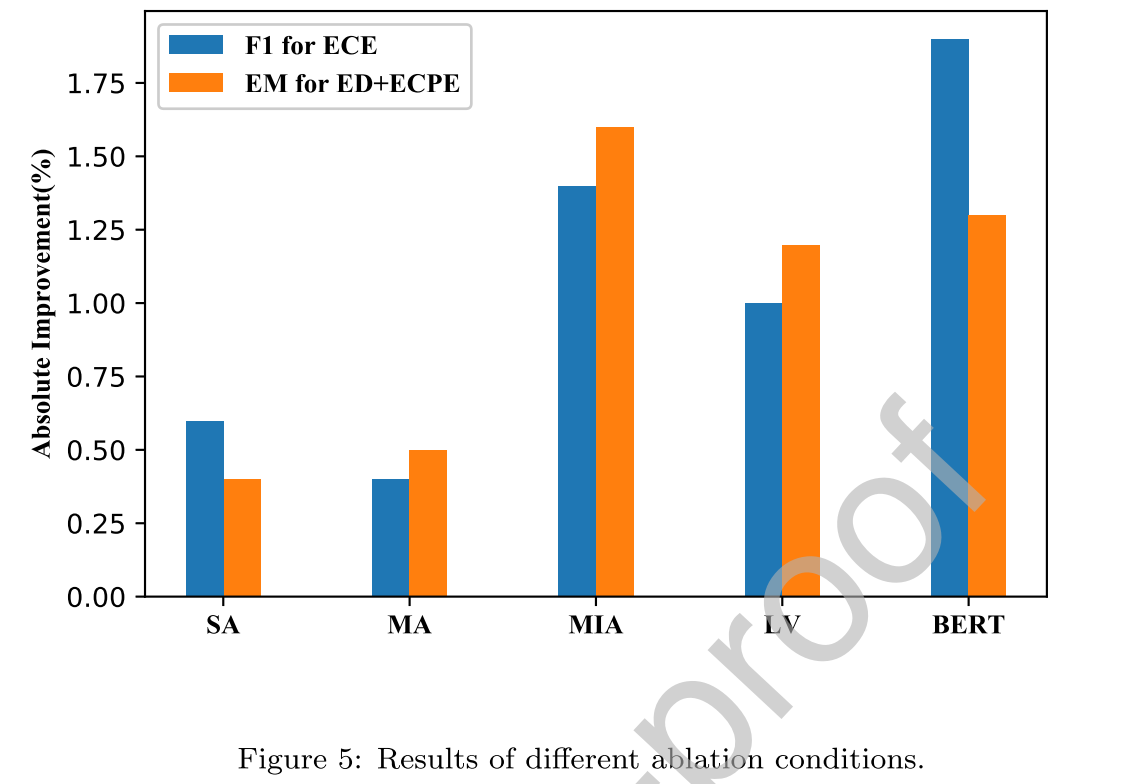## 5.7 定性分析